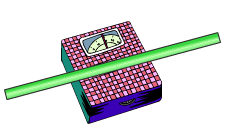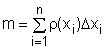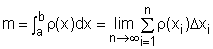Ch 6. Integrals Multimedia Engineering Math IndefiniteIntegral Area DefiniteIntegral FundamentalTheorem SubstitutionRule
 Chapter 1. Limits 2. Derivatives I 3. Derivatives II 4. Mean Value 5. Curve Sketching 6. Integrals 7. Inverse Functions 8. Integration Tech. 9. Integrate App. 10. Parametric Eqs. 11. Polar Coord. 12. Series Appendix Basic Math Units Search eBooks Dynamics Fluids Math Mechanics Statics Thermodynamics Author(s): Hengzhong Wen Chean Chin Ngo Meirong Huang Kurt Gramoll ©Kurt GramollMATHEMATICS - CASE STUDY IntroductionRod on Scale High school students in a physics class are asked to measure the mass of a non-homogeneous rod where the density varies along its length. What is known: The density of the rod can be modeled as       ρ(x) = x2 - x + 3 where x is the length. The density function, ρ(x), has units of g/m. The length of the rod is 4 m. Questions Determine the mass of the rod. Approach If the rod is divided into many small pieces, the total mass of the stick can be approximated by summing the mass of each piece.The definite integral can be used if the number pieces became infinite